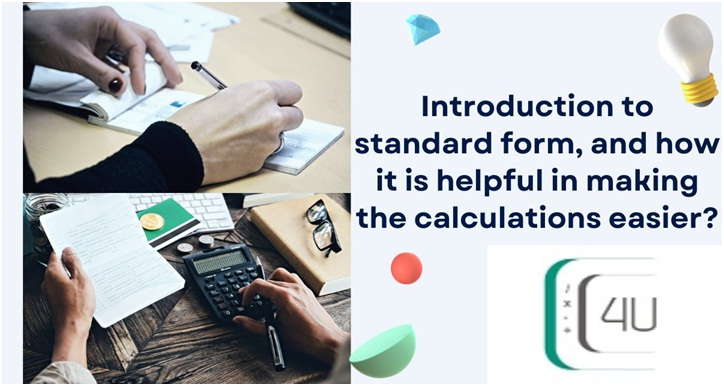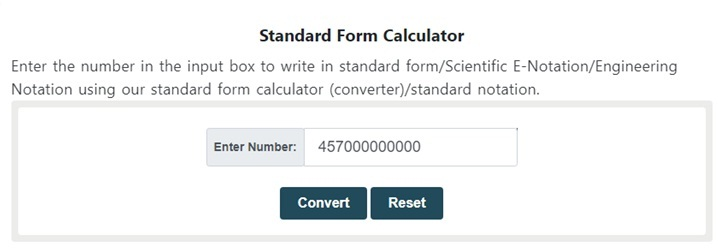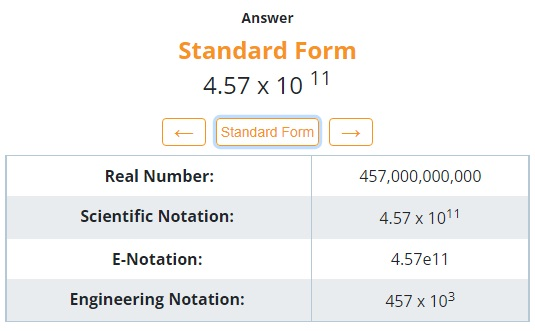# Introduction to standard form, and how it is helpful in making the calculations easier?The standard form is a commonly used term in daily sciences. This term plays a massive role in daily life calculations. It helps us to convert larger quantities or values into smaller numbers. Most of the time in physics and mathematics we got a number that contains more than 15 digits, it will occupy a lot of space.

The standard form helps us to write these large numbers into smaller numbers. So that it is beneficial as it occupies less space. This term refers to converting a number into the simplest form. Standard form plays different roles in different subjects.

In mathematics, it refers to converting numbers into generalized form and writing equations in canonical form. Scientists use the standard form to compare the magnitude of the quantities. In chemistry, it is very useful as it makes calculations easier for the students.

In this article, we will learn the definition of standard form, and the rules of converting a number into the simplest form with the help of examples.

## Definition of the standard form:

“In mathematics, a standard form of a mathematical object is a standard way of presenting that object as a mathematical expression. Often, it provides the simplest representation of an object and allows it to be identifieduniquely.”

## A glimpse from the 9th century:

The term standard form was firstly introduced by Muhammad Al-Khwarizmi in the early 9th century. He was a Persian mathematician. Scientific notation is another name of standard form, depending on the country in which you live.

In the United States of America, people use the term scientific notation whereas the British use the conventional word “standard form.” It has different names like normal form, canonical form, etc.

Now let's have a look at the writing techniques of the standard form.

## Method to write numbers in standard form

The method of writing a number into its standard form consists of some basic steps. By following these easy steps you will be able to convert the large numbers easily.

• Write the number i.e. 0.0000026
• Check the position of the decimal point.
• After checking the position move it to the right side of the 1st non-zero digit.
• After moving, count the number of digits you moved and multiply by “raise to power 10”
• If the decimal point is moved from left to right then the exponent will be negative but on the other hand, if it is moved in the opposite direction then the exponent will be positive.

### Example section:

The below examples will help you to understand the concept more clearly.

#### Example 1:

Convert 0.000000019 into the standard form

Solution:

Step 1: Write the number.

= 0.000000019

Step 2: Check the position of the decimal point

=0.000000019

Step 3: Move the decimal point to the right side of the 1st non-zero digit that is

= 1.9

Step 4: Now, count the number of digits you have moved and multiply the number with “raise to power 10”. As we have moved the decimal 8 points to the right side.

= 1.9× 10-8

Note: The exponent is negative because we have moved the decimal point towards the right side.

#### Example 2:

Convert 457000000000 into the standard form

Solution:

Possible intermediate steps are given below.

Step 1: Write the number.

= 457000000000.

Step 2: Check the exact position of the decimal point.

=457000000000.

Note: If there is no decimal point then it should be on most right side of the number

Step 3: Move the point to the right side of the 1st non-zero digit that is

= 4.57

Step 4: Now, count the digits you have moved and multiply the number with “raise to power 10”. As we have moved the decimal 11 points to the left side.

=4.57 × 1011

Note:The exponent is positive because we have moved the decimal point towards the left side.

A standard form calculator can help you to convert the numbers instantaneously to avoid calculations.As it gives the accurate answer without taking much time.#### Steps to use standard form calculator:

a. Enter the number
b. Click on convert and see the result#### Example 3:

Convert 1237974.675 × 105 into the standard form.

Solution:

Possible intermediate steps are given below.

Step 1: Write the number.

= 1237974.675 × 105

Step 2: Check the position of the decimal point.

=1237974.675 × 105

Step 3: Move the decimal point to the right side of the 1st non-zero digit that is

= 1.237974675

Step 4: Now, count the number of digits you have moved and multiply the number with “raise to power 10”. As we have moved the decimal 7 points to the left side.

= 1.237974675 × 105 × 107

= 1.237974675 × 105+7

=1.237974675 × 1012

#### Example 4:

Convert 974920.322 × 10-3 into the standard form.

Solution:

Possible intermediate steps are given below.

Step 1: Write the number.

= 974920.322 × 10-3

Step 2: Check the position of the decimal point.

=974920.322× 10-3

Step 3: Move the decimal point to the right side of the 1st non-zero digit that is

= 9.74920322

Step 4: Now, count the number of digits you have moved and multiply the number with “raise to power 10”. As we have moved the decimal 5 points to the left side.

= 9.74920322 × 10-3 × 105

= 9.74920322× 10-3 + 5

= 9.74920322× 102

### Summary:

In this article, we have studied the basic definition of the standard form, its uses, and its basic history. We explained this topic with the help of examples. You witnessed that it is not a difficult topic. Now you can solve all the basic problems related to this topic within no time.